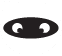# Relationship between Margins and Markups

Relationship between Markup / Margin:

Margin = Profit / Revenue
Margin = Markup / (1 + Markup)
Markup = Margin / (1 - Margin)

Margin of 12% on \$100 means \$88 Ad Spend + \$12 in pocket
Markup of 12% on \$100 means \$100 Ad Spend + \$12 in pocket

Both give the same \$12 in your pocket, but with margins the advertiser spends \$100 and with a Markup the advertiser spends \$112

Formula for calculating cost with a Markup:
Total Cost to Advertiser = Actual Cost ( 1 + Markup)

(Inverted:)
Actual Cost = Total Cost to Advertiser / ( 1 + Markup)

Formula for calculating cost with a Margin:
Total Cost to Advertiser = Actual Cost / (1 - Margin)

(Inverted:)
Actual Cost = Total Cost to Advertiser * (1 - Margin)